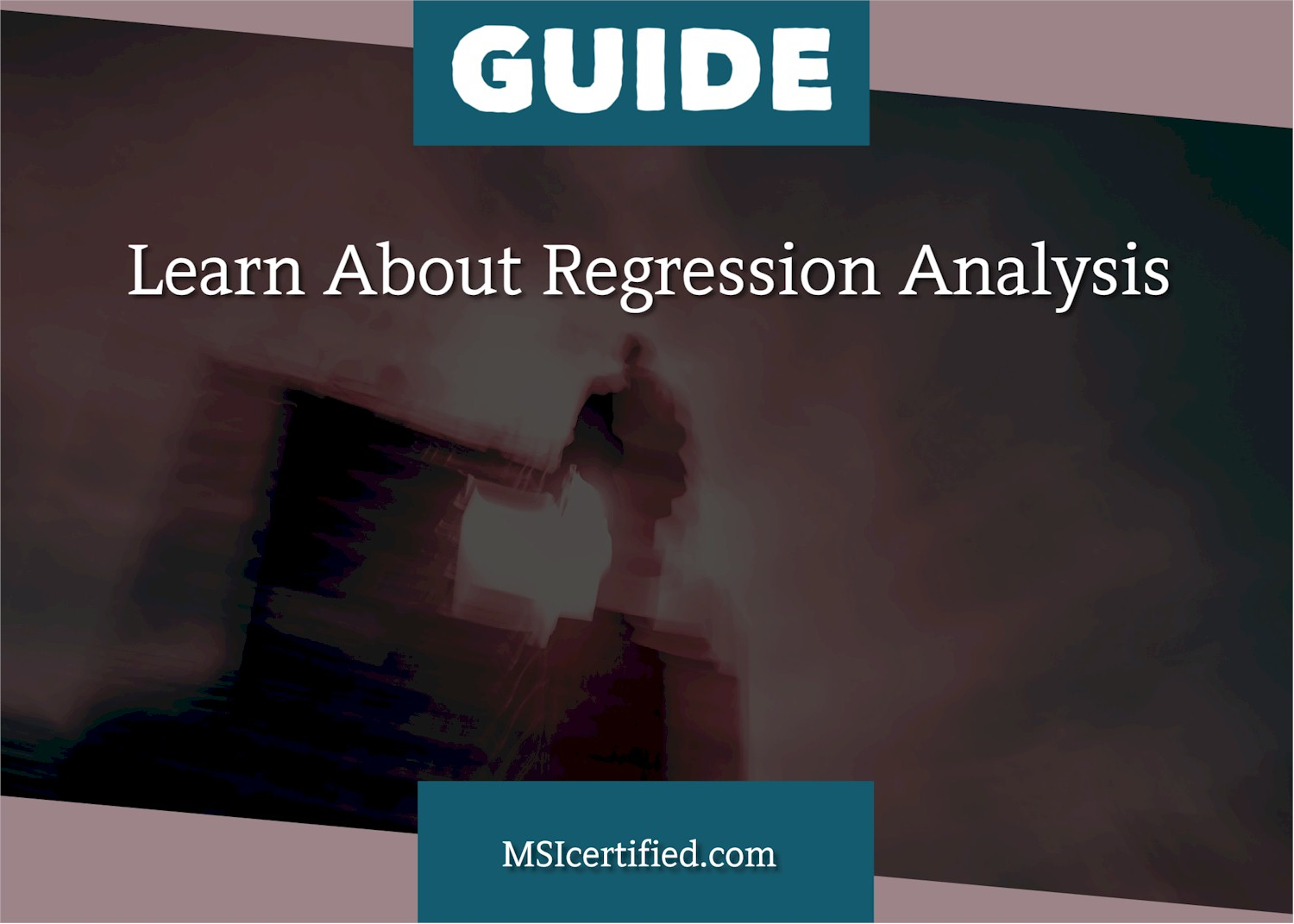# Guide to Regression AnalysisRegression analysis is a statistical technique that helps to identify the relationship between a dependent variable and one or more independent variables.  This method can be used to analyze the relationship between two or more variables and to predict the values of one variable based on the values of the other variables.  Regression analysis is widely used in fields such as economics, finance, biology, and social sciences.  It is popular among certified Six Sigma professionals and used in Six Sigma projects.

Today we will cover the basics of regression analysis, including its types, assumptions, and steps to perform regression analysis.

## Types of Regression Analysis:

There are two main types of regression analysis: simple linear regression and multiple linear regression.

Simple linear regression involves two variables: one variable is dependent, and the other is independent.  The objective is to find the best-fit line representing the relationship between the two variables.  The best-fit line is the one that minimizes the sum of the squared errors between the predicted values and the actual values.

Multiple linear regression involves more than two variables, one dependent variable, and the other independent variables.  In this case, the objective is to find the best-fit plane that represents the relationship between the dependent variable and the independent variables.  The best-fit plane is the one that minimizes the sum of the squared errors between the predicted values and the actual values.

## Assumptions of Regression Analysis:

Before performing regression analysis, certain assumptions must be met.  These assumptions include:

1. Linear Relationship: A linear relationship between the independent and dependent variables must exist.
2. Independence: The observations must be independent of each other.
3. Homoscedasticity: The variance of the errors must be constant across all values of the independent variables.
4. Normality: The errors must be normally distributed.

## Steps to Perform Regression Analysis:

1. Define the Problem: The first step is to define the problem and identify the variables that will be used in the analysis.
2. Collect the Data: Collect data on the variables of interest.
3. Check for Outliers: Identify and remove outliers, as they can skew the results of the analysis.
4. Check for Linearity: Check for linearity between the dependent and independent variables.
5. Check for Homoscedasticity: Check for homoscedasticity by plotting the residuals against the predicted values.
6. Check for Normality: Check for normality by plotting the residuals against a normal distribution.
7. Run the Regression Analysis: Once the assumptions have been met, run the regression analysis and examine the results.
8. Interpret the Results: Interpret the results and determine the significance of the independent variables.
9. Make Predictions: Use the results to make predictions about the dependent variable.

## Conclusion:

Regression analysis is a powerful statistical tool that can be used to identify the relationship between two or more variables and make predictions about the dependent variable.  By understanding the types, assumptions, and steps involved in regression analysis, you can perform this analysis and use the results to make informed decisions in various fields.  Remember to check for the assumptions and interpret the results carefully to ensure that the analysis is accurate and reliable.